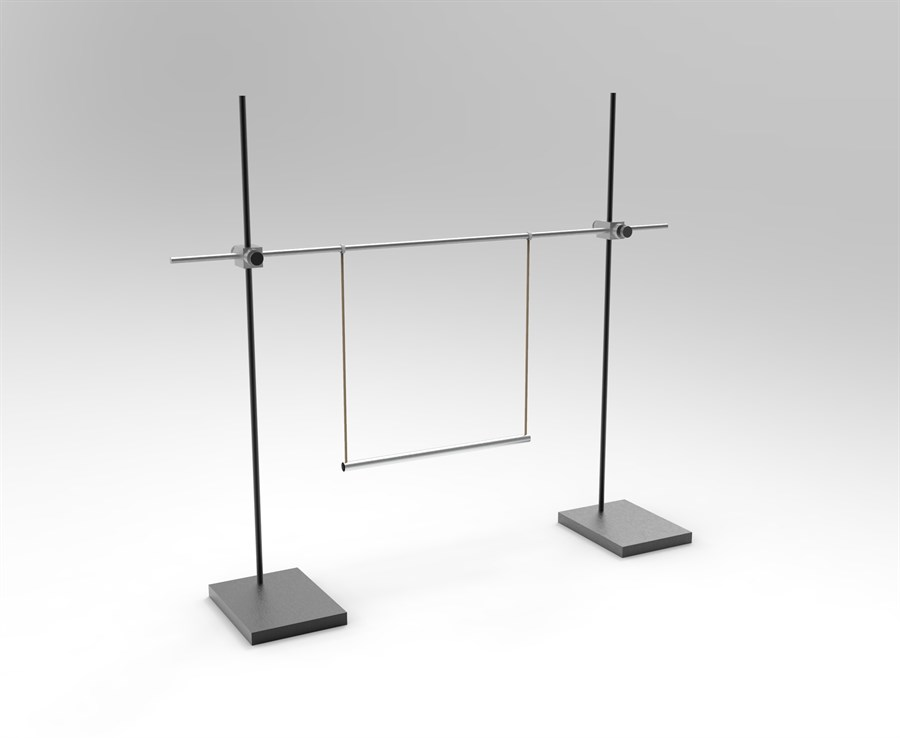# Doubly Suspended Pendulum

Physics of a complex pendulum.
Investigate Newton’s second law for angular motion.
Determine the moment of inertia for a bifilar pendulum.
Mathematical modeling of forces.
Calculating uncertainty and graphical representation of data.
Product Code: bifilar pendilum
Brand: Qosain Scientific
Availability: In Stock

Rs. 50,000
How does it Work?

How does it work?A bifilar pendulum is a straight rod, hanging horizontally from two parallel wires, called “filars”, which allow the rod to rotate freely about          the vertical axis. In the stationary condition, the filars stay vertically and there are no forces in other directions. As soon as the pendulum is twisted, the              rods move along with it and the horizontal components of the tension in the filars creates a torque, forcing the rod to accelerate back towards the starting          position. This torque is proportional to the angle of twist and, just like a simple pendulum, causes the rod to start oscillating about the vertical axis.                      Moment of inertia plays the same role as inertial in the simple pendulum.

Using Newton’s second law of motion for angular motiona relationship between toque, moment of inertia and angular acceleration is found. This can                  further be used to find the period of a bifilar pendulum which is also measured using the setupThe model is further used to investigate the uncertainities in        the measurement which is a crucial part of the investigation.

Parts Included
§          Clamps
§          Lab stands
§          Pendulum rod
§          Filar wires
§          Stopwatch
No Specifications Found